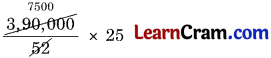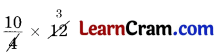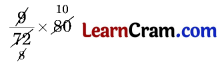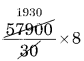# DAV Class 6 Maths Chapter 4 Worksheet 3 Solutions

The DAV Class 6 Maths Book Solutions Pdf and DAV Class 6 Maths Chapter 4 Worksheet 3 Solutions of Ratio, Proportion and Unitary Method offer comprehensive answers to textbook questions.

## DAV Class 6 Maths Ch 4 WS 3 Solutions

Question 1.
Sahil buys 8 books for ₹ 72. What is the cost of 45 books?
Solution:
Cost of 8 books = ₹ 72
Cost of 1 book = ₹ $$\frac{72}{8}$$
Cost of 45 books = ₹ $$\frac{72}{8}$$ × 45
= ₹ 9 × 45 = 405
Hence, the required cost = ₹ 405.

Question 2.
The bus fare for 20 persons from Delhi to Chandigarh is ₹ 9500. Find the bus fare for 19 persons.
Solution:
Bus fare for 20 persons = ₹ 9500
Bus fare for 1 person = ₹ $$\frac{9500}{20}$$
Bus fare for 19 persons = ₹= ₹ 475 × 19
Hence, the required Bus fare = ₹ 9025.Question 3.
Arun earns ₹ 3,90,000 per year. Find his earnings for 25 weeks.
Solution:
1 year = 4 × 13 = 52 weeks
Earnings for 48 weeks = ₹ 3,90,000
Earnings for 1 week = ₹ $$\frac{3,90,000}{52}$$
Earnings for 25 weeks
= ₹= 7500 × 25
= ₹ 1,87,500
Hence, the required cost = ₹ 1,87,500.

Question 4.
The cost of 18 kg wheat is ₹ 216. Find the cost of 1 quintal wheat.
Solution:
1 quintal = 100 kg
Cost of 18 kg of wheat = ₹ 216
Cost of 1 kg of wheat = ₹ $$\frac{216}{18}$$
Cost of 100 kg of wheat
= ₹= ₹ 12 × 100
= ₹ 1200
Hence, the required cost = ₹ 1200.Question 5.
Four erasers are purchased for ₹ 10. Find the cost of 1 dozen erasers.
Solution:
Cost of 4 erasers = ₹ 10
Cost of 1 eraser = ₹ $$\frac{10}{4}$$
Cost of 12 erasers
= ₹= ₹ 10 × 3
= ₹ 30
Hence, the required cost = ₹ 30.

Question 6.
A worker earns ₹ 18,000 in 15 months,

(a) How much will the worker earn in 7 months?
Solution:
Earning in 15 months = ₹ 18000
Earning in 1 month = ₹ $$\frac{18000}{15}$$
Earning in 7 months
= ₹= ₹ 1200 × 7
= ₹ 8400
Hence, the required earning = ₹ 8400.

(b) In how many months will he earn ₹ 3,600?
Solution:
₹ 18,000 are earned in 15 months
₹ 1 is earned in $$\frac{15}{18000}$$ months
₹ 3600 are earned in
=months
= 3 months
Hence, the required time = 3 months.Question 7.
The weight of 72 books is 9 kg:

(a) Find the weight of 80 such books.
Solution:
Weight of 72 books = 9 kg
Weight of 1 book = $$\frac{9}{72}$$ kg
Weight of 80 books
== 10 kg
Hence, the required weight = 10 kg.

(b) How many books will weigh 6 kg?
Solution:
9 kg is the weight of 72 books
1 kg is the weight of $$\frac{72}{9}$$ books
6 kg is the weight of $$\frac{72}{9}$$ × 6 = 48 books
Hence, the required number of books = 48.

Question 8.
A factory produced 57,900 screws in the month of April, 2015 (The factory produces same number of screws everyday).

(a) How many screws did the factory produce in 8 days?
Solution:
Production of screws in 30 days = 57,900
Production of screws in 1 day = $$\frac{57900}{30}$$
Production of screws in 8 days
== 1930 × 8
= 15440 screws
Hence, the required number of screws = 15440.

(b) In how many days, did the factory produce 34,740 screws?
Solution:
57900 screws are produced in 30 days
1 screw is produced in $$\frac{30}{57900}$$ days
34740 screws are produced in
== 18 days
Hence, required number of days = 18.### DAV Class 8 Maths Chapter 3 Value Based Questions

Question 1.
Ramesh went to the market to buy potatoes. He found a shopkeeper selling 2 1/2 kg potatoes for ₹ 30. Ramesh bought 15 kg potatoes from him and gave the shopkeeper ₹ 500 note. Shopkeeper gave back a balance of ₹ 350 to Ramesh. On way back home he realised that the shopkeeper had returned him more money. Ramesh went back to the shop and returned the extra amount.

(a) What was the cost of 15 kg potatoes?
Solution:
Cost of 2 1/2 kg potatoes = ₹ 30
or cost of 5/2 kg potatoes = ₹ 30
∴ cost of 1 kg potatoes = ₹ 30 ÷ 5/2
= ₹ 30 × 2/5 = ₹ 12
∴ cost of 15 kg potatoes = ₹ 12 × 15 = ₹ 180

Solution:
Ramesh returned to the shopkeeper = ₹ 180 – ₹ 150 = ₹ 30

(c) What value is exhibited by Ramesh’s act?
Solution:
Honesty.Question 2.
Mehak, Harpreet and Raj planted trees in their school and its neighbourhood during the Vanamahotsav Celebration. They were really very happy and excited to be a part of the celebrations. Mehak planted 18 trees, Harpreet planted 24 trees and Raj planted 32 trees.

(a) What is the ratio of trees planted by Mehak to those planted by Harpreet?
Solution:
No. of trees planted by Mehak = 18
No. of trees planted by Harpreet = 24
∴ Ratio of trees planted by Mehak to Harpreet = 18 : 24 = 3 : 4

(b) How many more trees should Raj plant so that the ratio of trees planted by Mehak to that of Raj become 1 : 2?
Solution:
Let Raj have to plant n more trees
∴ $$\frac{18}{(32+n)}=\frac{1}{2}$$
or 36 = 32 + n or
n = 4
∴ Raj have to plant 4 more trees.

(c) How do you celebrate Vanamahotsav in your school? Why should we take care of our environment?
Solution:
By planting more trees.